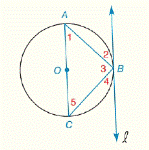Chapter 6.CR, Problem 12CR### Elementary Geometry for College St...

6th Edition
Daniel C. Alexander + 1 other
ISBN: 9781285195698

#### Solutions

Chapter
Section### Elementary Geometry for College St...

6th Edition
Daniel C. Alexander + 1 other
ISBN: 9781285195698
Textbook Problem
3 views

# G i v e n :           ⨀ O   w i t h   t a n g e n t   l   a n d   m ∠ 5 = 40 °           F i n d :             m ∠ 1 ,     m ∠ 2 , m ∠ 3 ,     m ∠ 4 ,To determine

To find: m1,m2, m3, m4

Explanation

Theorem:

1) An angle inscribed in a semicircle is a right angle.

2) The measure of an angle formed by a tangent and a chord drawn to the point of tangency is one-half the measure of the intercepted arc.

3) The measure of an inscribed angle of a circle is one-half the measure of its intercepted arc.

Calculation:

Given that

m5=40° and l is tangent to the circle at point B.

By theorem, An angle inscribed in a semicircle is a right angle.

m3 is inscribed in a semicircle.

So, m3=90°.

Since, sum of the three angles in a triangle is 180°

In ABC, m1+m5+m3=180°

Let m5=40°,m3=90°

40°+m1+90°=180°

m1+130°=180°

Subtract 130°, m15+130°-130°=180°-130°

So, m1=50°

And m1=50° is the angle intercepted by the arc AB^

### Still sussing out bartleby?

Check out a sample textbook solution.

See a sample solution

#### The Solution to Your Study Problems

Bartleby provides explanations to thousands of textbook problems written by our experts, many with advanced degrees!

Get Started

#### 14. If , find n.

Mathematical Applications for the Management, Life, and Social Sciences

#### Evaluate the limit, if it exists. limu24u+13u2

Single Variable Calculus: Early Transcendentals, Volume I

#### True or False: converges.

Study Guide for Stewart's Multivariable Calculus, 8th